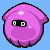### Science Quiz

Random Science or Numbers Quiz

# Fun With 1234 Quiz Stats

#### bytobley  Plays Quiz Updated Mar 6, 2020

Score 0/20 Timer 06:00
0 Plays Today
Equation Order % Correct
_ ⁄ _ – _ + _ = 04 ⁄ 2 – 3 + 1
94.2%
_ * _ – _ ⁄ _ = 13 * 1 – 4 ⁄ 2
67.2%
_ – _ + _ * _ = 12 – 4 + 3 * 1
61.8%
_ + _ ⁄ _ – _ = 13 + 2 ⁄ 1 – 4
45.4%
_ + _ – _ ⁄ _ = 21 + 3 – 4 ⁄ 2
41.5%
_ * _ + _ – _ = 31 * 4 + 2 – 3
38.2%
_ * _ ⁄ _ – _ = 22 * 3 ⁄ 1 – 4
36%
_ ⁄ _ + _ * _ = 54 ⁄ 2 + 1 * 3
30.9%
_ – _ ⁄ _ + _ = 34 – 3 ⁄ 1 + 2
30.1%
_ – _ * _ + _ = 53 – 1 * 2 + 4
29%
Equation Order % Correct
_ – _ + _ ⁄ _ = 43 – 1 + 4 ⁄ 2
27.5%
_ ⁄ _ * _ + _ = 143 ⁄ 1 * 4 + 2
21.8%
_ ⁄ _ * _ + _ = 73 ⁄ 2 * 4 + 1
21.4%
_ + _ * _ – _ = 61 + 2 * 4 – 3
20.6%
_ ⁄ _ * _ – _ = 104 ⁄ 1 * 3 – 2
20.1%
_ * _ – _ + _ = 92 * 3 – 1 + 4
19.8%
_ – _ + _ * _ = 132 – 1 + 3 * 4
17.4%
_ * _ ⁄ _ + _ = 112 * 4 ⁄ 1 + 3
15.6%
_ + _ ⁄ _ * _ = 104 + 3 ⁄ 1 * 2
15.3%
_ + _ * _ ⁄ _ = 113 + 4 * 2 ⁄ 1
14.8%

## From the Vault See Another

Typing Challenge: 100 'D' Words
This quiz is delightful, delicious, and de-lovely.